# 回溯算法介绍

1. 从根节点出发深度搜索解空间树
2. 搜索到有解的分支时，继续向下搜索
3. 搜索到无解的分支时，回退到上一步，顾名思义“回溯”

# 框架套路

talk is cheap，show you the 套路，框架如下

结果集=[]
function dfs(选择列表，已选择的数组)
if 结束条件
结果集追加
return
for 选择 in 选择列表
做选择
dfs(选择列表, 已选择数组) 进入下一次选择
取消选择
dfs(选择列表，[])
return 结果集


1. 选择列表。当前可以做出的选择
2. 已选择路径。已经做出的选择
3. 结束条件。无法再做出选择的条件

# 算法示例

## 全组合

aa
ab
ba
bb

import copy

# 全组合
def combination(str_list):
res = []

max_len = len(str_list)

def dfs(str_list, track_list):
if len(track_list) == max_len:  # 满足条件，加入结果集
res.append(track_list)
return
for c in str_list:
track_list.append(c)  # 选择
dfs(str_list, copy.copy(track_list))  # 进入下一次选择
track_list.pop()  # 取消选择

dfs(str_list, [])
return res


1. 选择列表。可以选择的字符串，比如['a','b','c']，对应变量str_list。
2. 已选择路径。已经做出的选择，比如已经选择了['a']，对应变量track_list。
3. 结束条件。无法再做出选择的条件，已选择的数组长度等于最大长度，对应len(track_list) == max_len

for v in combination(['a', 'b']):
print(v)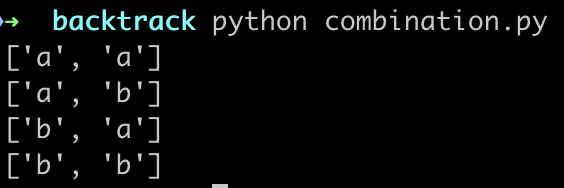## 全排列

import copy

# 全排列
def permute(str_list):
res = []

max_len = len(str_list)

def dfs(str_list, track_list):

if len(track_list) == max_len:  # 满足条件，加入结果集
res.append(track_list)
return
for c in str_list:
if c in track_list:  # 已经存在的不再添加
continue
track_list.append(c)  # 选择
dfs(str_list, copy.copy(track_list))  # 进入下一次选择
track_list.pop()  # 取消选择

dfs(str_list, [])
return res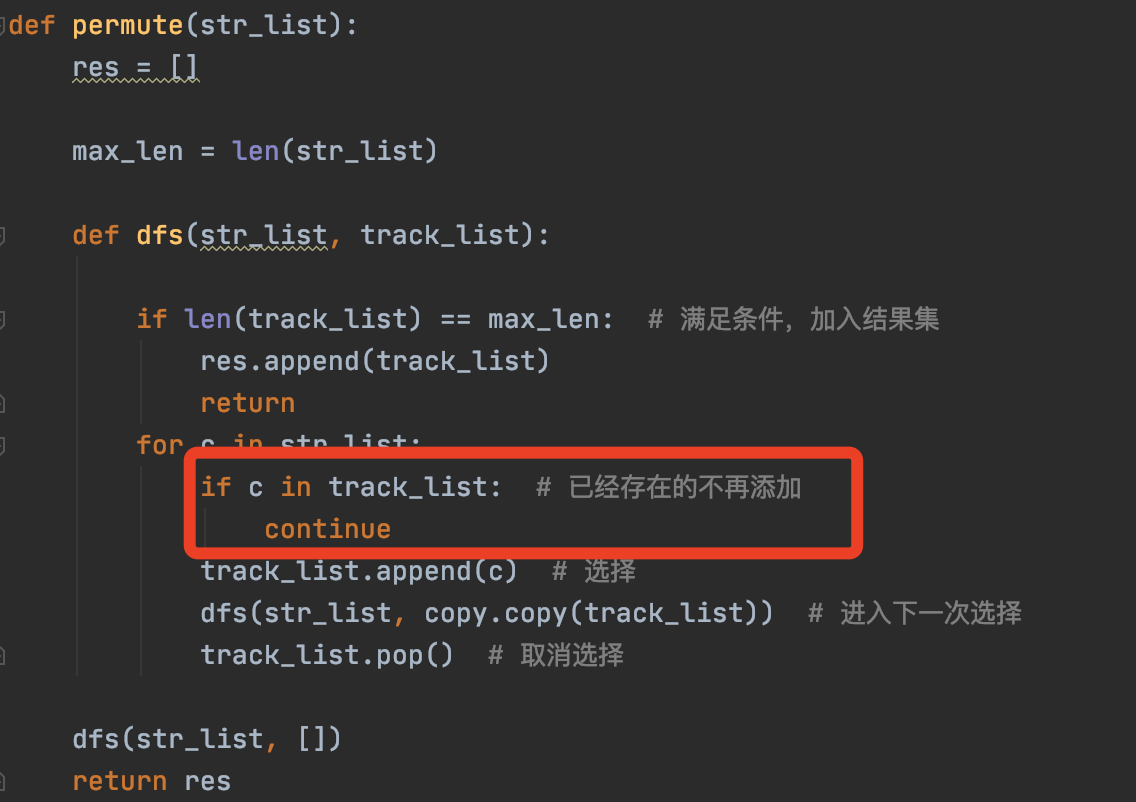for v in permute(['c', 'q', 'h']):
print(v)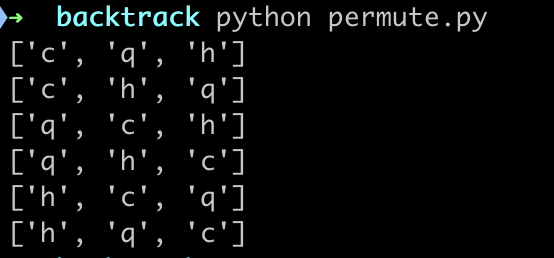## 凑零钱

def coin_change(coins, amount):
res_list = []

def dfs(n, track_list):
if n == 0:
res_list.append(track_list)  # 满足条件
return 0

if n < 0:
return -1

for coin in coins:
track_list.append(coin)  # 做选择
dfs(n - coin, copy.copy(track_list))  # 选择一个硬币，目标金额就会减少，解变为1+sub
track_list.pop()  # 取消选择

dfs(amount, [])
return res_list


1. 选择列表。可以选择的硬币，对应coins数组。
2. 已选择路径。已经做出的选择，对应track_list数组。
3. 结束条件。无法再做出选择的条件，金额为0和负的时候。

for v in coin_change([2, 3, 5], 10):
print(v)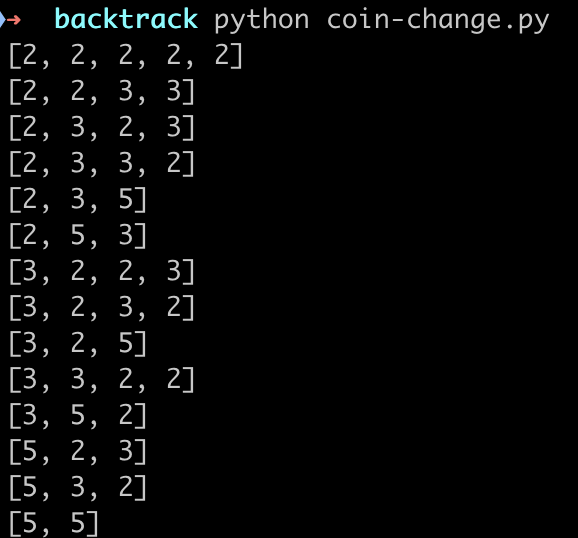## N皇后

第一种方案
. Q . .
. . . Q
Q . . .
. . Q .

. . Q .
Q . . .
. . . Q
. Q . .


# N皇后问题
def solve_n_queens(n):
res = []

def dfs(board, row):
if row == n:  # 到达最后一行，追加结果集
res.append(board)
for col in range(n):
# 排除不合法的选择
if not is_valid(board, row, col, n):
continue
board[row][col] = 'Q'  # 选择第row行第col列放Q

dfs(copy.deepcopy(board), row + 1)

board[row][col] = '.'  # 撤销选择
return False

board = [['.'] * n for _ in range(n)]  # 初始化二维数组
dfs(board, 0)  # 从第0行开始做选择
return res

# 判断是否能在board[row][col]放置Q
def is_valid(board, row, col, n):
# 垂直方向是否有Q
for v in range(row):
if board[v][col] == 'Q':
return False
# 左上方是否有Q
i, j = row - 1, col - 1
while i >= 0 and j >= 0:
if board[i][j] == 'Q':
return False
i = i - 1
j = j - 1
# 右上方是否有Q
i, j = row - 1, col + 1
while i >= 0 and j <= n - 1:
if board[i][j] == 'Q':
return False
i = i - 1
j = j + 1
return True


N皇后的解法是，在每行做选择，选择为N列，做出选择后，进入下一行继续做选择

1. 选择列表。可以选择的列，对应的是0-n的任意一列。
2. 已选择路径。已经做出的选择，对应board二维数组。
3. 结束条件。无法再做出选择的条件，也就是已经到达最后一行的时候。

res = solve_n_queens(8)
for data in res:
print('-' * 20)
for v in data:
print(" ".join(v))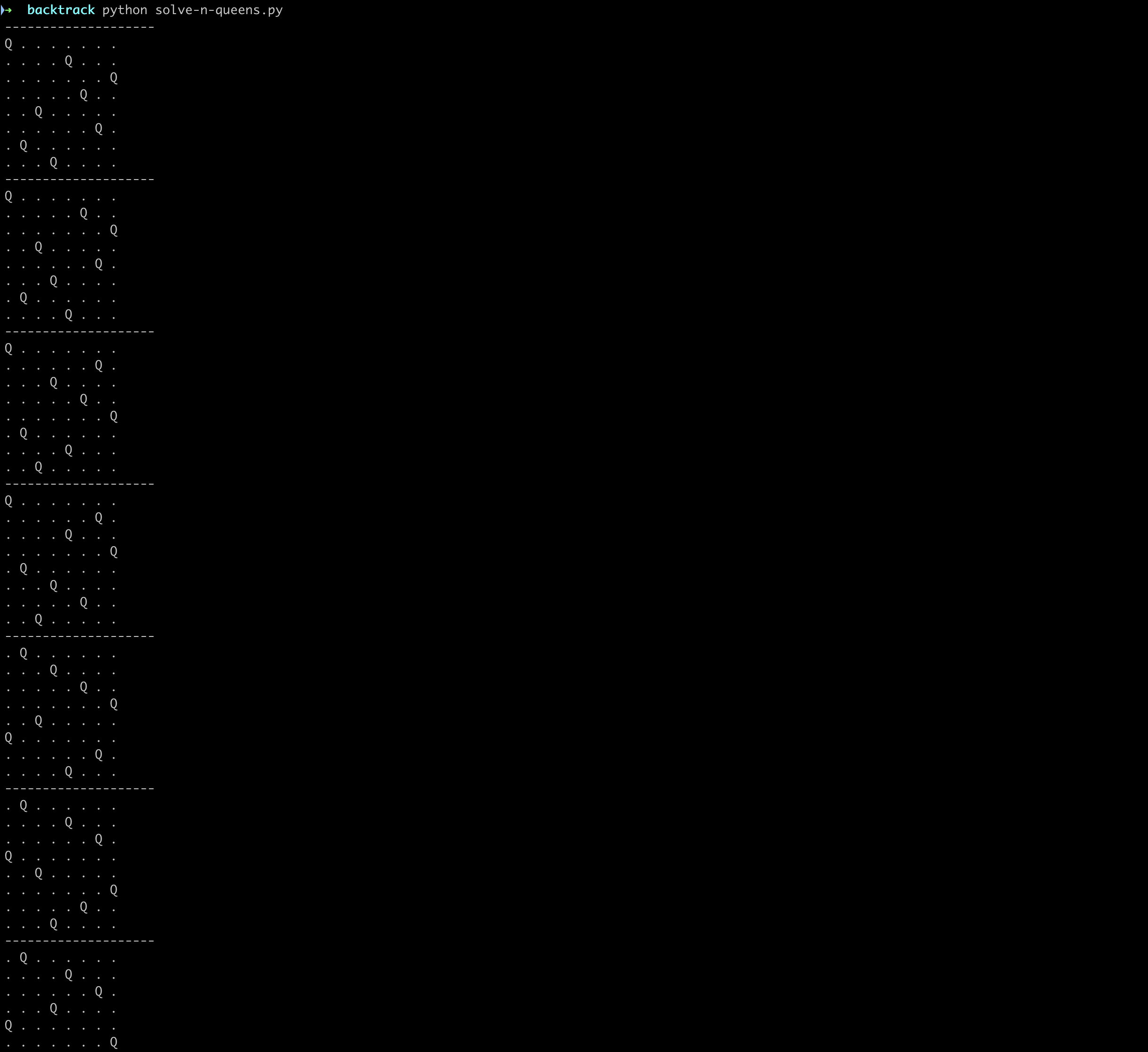## 最长递增子序列

import copy

def long_increasing_subsequence(arr):
res_list = []
n = len(arr)

max_len = 1
max_sub = []

# 从第i个元素做选择
def dfs(i, track_list):
# 到达末尾 或 下一个元素比track数组最后一个大
if i == n or (len(track_list) > 0 and arr[i] < track_list[-1]):
res_list.append(track_list)  # 满足条件
nonlocal max_len, max_sub
if max_len < len(track_list):
max_len = len(track_list)
max_sub = track_list
return
for v in range(i, n):
if len(track_list) > 0 and arr[v] < track_list[-1]:
continue
track_list.append(a[v])  # 做选择
if v < n:
dfs(v + 1, copy.copy(track_list))  # 下一次选择
track_list.pop()  # 取消选择

dfs(0, [])
return max_sub, res_list

a = [10, 9, 2, 5, 3, 7, 101, 18]
max_sub, res_list = long_increasing_subsequence(a)
print(max_sub)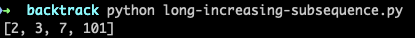## 最长公共子序列

import copy

def long_common_subsequence_all(str1, str2):
len1, len2 = len(str1), len(str2)
res_list = []

def dp(i, j, track1, track2):
if i == len1 or j == len2:
# 到头了，收集一下，相同的子序列
res_list.append("".join(track1))
return

c_track1 = copy.copy(track1)
c_track2 = copy.copy(track2)

if str1[i] == str2[j]:
# 找到一个lcs中的元素，str1和str2分别选中，继续往下找
c_track1.append(str1[i])
c_track2.append(str2[j])
dp(i + 1, j + 1, c_track1, c_track2)
return
else:
dp(i, j + 1, c_track1, c_track2)
dp(i + 1, j, c_track1, c_track2)

dp(0, 0, [], [])

lcs = ""
for cs in res_list:
if len(cs) > len(lcs):
lcs = cs

return lcs, res_list

s1 = "abcde"
s2 = "ace"
lcs, res_list = long_common_subsequence_all(s1, s2)
print(lcs)


# 优化思路

## 备忘录避免重复计算

def coin_change_optimization(coins, amount):
memo = {}
def dfs(n):
if n in memo:
return memo[n]
if n == 0:
return 0
if n < 0:
return -1

min_res = float('INF')
for coin in coins:
sub = dfs(n - coin)  # 选择一个硬币，目标金额就会减少，解变为1+sub
if sub == -1:
continue
if min_res > 1 + sub:  # 更新最小值
min_res = 1 + sub

memo[n] = min_res if min_res != float('INF') else -1
return memo[n]

return dfs(amount)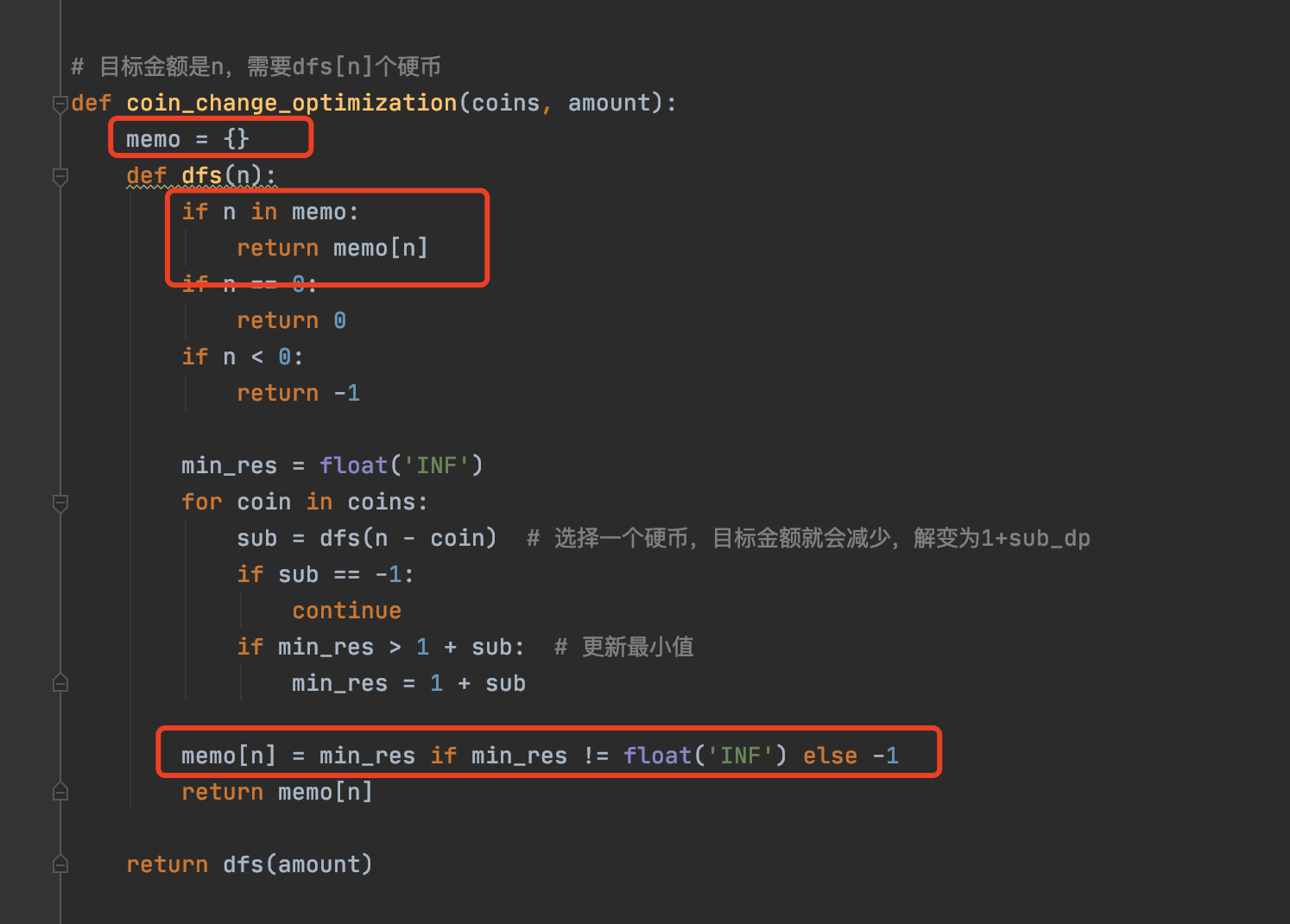## 向上返回阻断其他递归

# N皇后问题
def solve_n_queens(n):
res = []

def dfs(board, row):
if row == n:  # 到达最后一行，追加结果集
res.append(board)
return True
for col in range(n):
# 排除不合法的选择
if not is_valid(board, row, col, n):
continue
board[row][col] = 'Q'  # 选择第row行第col列放Q

if dfs(copy.deepcopy(board), row + 1):
return True

board[row][col] = '.'  # 撤销选择
return False

board = [['.'] * n for _ in range(n)]  # 初始化二维数组
dfs(board, 0)  # 从第0行开始做选择
return res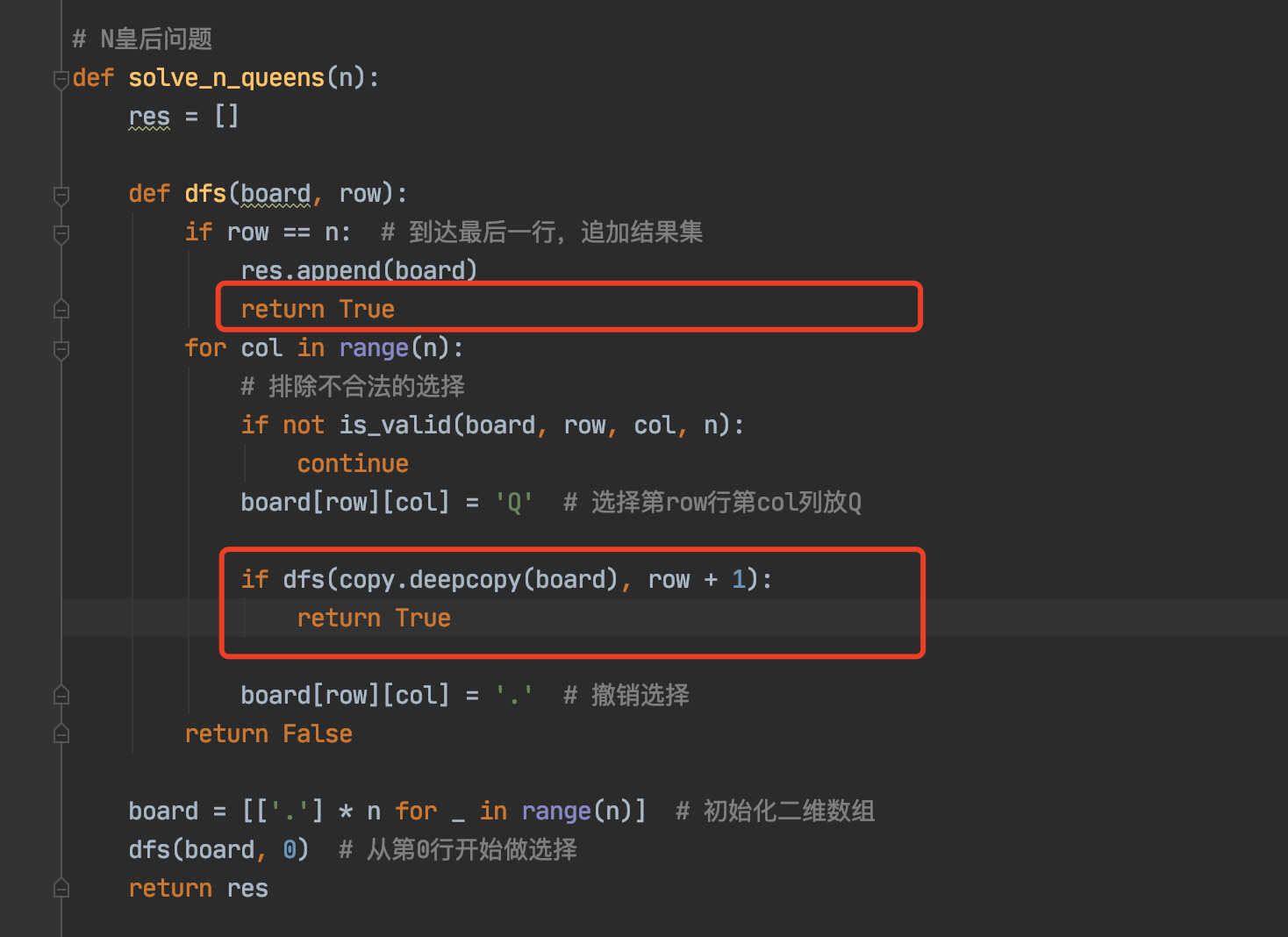posted @ 2021-08-24 11:49  雪山飞猪  阅读(311)  评论(0编辑  收藏  举报